# 2. Vector Kinematics

## Introduction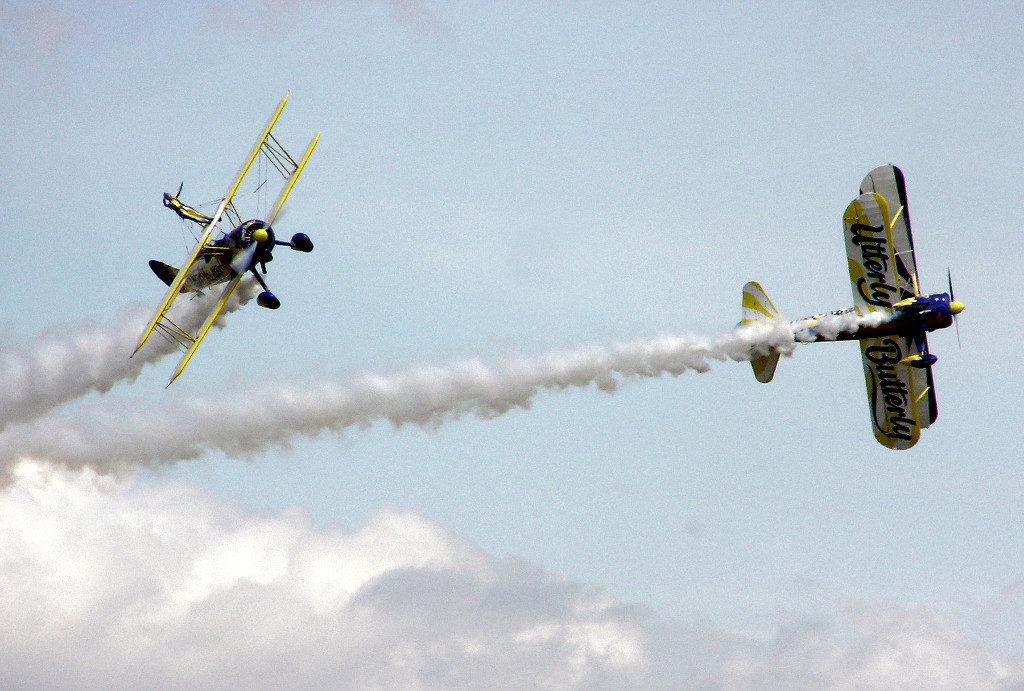When an object can move freely in space, without following a particular path, its position can no longer be described with one single variable as in the examples given in the previous chapter. In the seventeenth century, the mathematician Gottfried Leibniz wrote that it would be useful to create an area of mathematics that described positions directly, in the same way that variables are used in algebra to represent numerical values. In the same century Isaac Newton stated the parallelogram rule to add forces. However, the concept of vector used today, turning the dream of Leibnitz a reality, was only invented many years later in the nineteenth century.

## 2.1. Vectors

A quantity that has the same value when measured by different observers in different reference frames is called a scalar. Some of the quantities used in the previous chapter are scalars; for example, the displacement and the time interval .

Examples of physical quantities which are not scalar are the components of the position, velocity and acceleration along an axis. Changing the direction or origin of that axis, the values ​​of these quantities also change.

It is useful to write the equations of physics so that they are equal in any reference frame and vectors can be used to achieve this goal. A typical example is the displacement vector, which is a directed line segment between two points P1 and P2 in space, where the first point is considered the initial point, or origin, of the segment and the second point is the terminal point of the segment.

For example, in Figure 2.1, represents the vector starting at P1 and ending at P2; the arrow in P2 indicates that it is the end point and an arrow is also used on top of the letter used to denote the vector, to make it clear that this is a vector and not a regular algebraic variable.

### 2.1.1. Vector properties

The distance between the initial and terminal points of a displacement vector is called its magnitude or length. In this book, if a vector is denoted by its magnitude is then denoted by (the same letter without an arrow on top of it). Since the distance between two points is a scalar, the magnitude of a vector is a scalar too. A vector is defined by its magnitude and its direction, which is the orientation of the line through the two points.

Two vectors are equal if, and only if, they have the same direction and magnitude regardless of their initial point. For example, in Figure 2.1 the vector between points P1 and P2 is equal to the vector between P3 and P4 and that is why they were both denoted by . The distance between P3 and P4 equals the distance between P1 and P2 and the lines going through those two pairs of points are parallel. Vector , from point P5 to P6, is not equal to because its magnitude and direction are different. This kind of vectors in which the initial point does not matter, are called free vectors.

In Figure 2.2, starting from the point P the displacement vector ends at point Q and then from that point the displacement vector leads to the final point R. Namely, the combined displacements and are equivalent to a displacement from point P to R, denoted in the figure by vector . The combined displacement defines the sum of vectors and

(2.1)

Therefore, the addition of two vectors is done by placing the initial point of the second vector on the terminal point of the first one and then joining the initial point of the first vector with the terminal point of the second one.

The equation + = implies that = and Figure 2.2 shows that vector joins the terminal point of with the terminal point of , when those two vectors are placed on the same initial point. Thus, the subtraction of two vectors is done placing those two vectors on the same initial point and then joining the terminal point of the second vector to the terminal point of the first vector.

The addition of vectors is a commutative operation: placing the initial point of vector on the terminal point of vector leads to the same combined vector obtained by placing the initial point of vector on the terminal point of vector (see Figure 2.3). The sum of vectors and is the diagonal of the parallelogram formed by placing the vectors on a common initial point and then repeating each vector with its initial point on the terminal point of the other. The addition of several vectors also follows the associative law.

According to the definition of vector addition and subtraction, the sum of a vector with itself, + , is a vector with the same direction but magnitude twice as big. Subtracting a vector from itself, - , leads to the null vector (a vector whose initial and terminal points are the same). Generalizing these results, the product of a scalar and a vector , is defined as another vector in the same direction, if is positive, or in the opposite direction if is negative and with magnitude equal to . The product of a scalar and a vector is usually written with the scalar first, followed by the vector, but the order does not really matter. If is equal to zero, then is the null vector .

Any vector is equal to the product of its magnitude, , and a unit vector , with the same direction of but magnitude equal to 1 (see Figure 2.4). Thus, the unit vector defines the direction of the corresponding vector . In this book unit vectors are denoted by a letter with a hat, instead of an arrow, on top of it.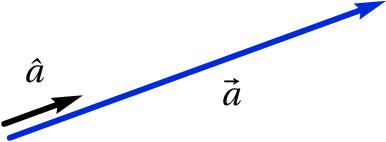Figure 2.4: Unit vector ˆ a defining the direction of vector  a .

As mentioned in the previous chapter, the position of a point P in space can be given by three coordinates in some system of coordinates and the rectangular coordinates system was introduced. Figure 2.5 shows the rectangular coordinates ( , , ) of point P.

There are two different ways to define the directions of the three axes , e . The usual way to define those directions follows the right-hand rule: after closing the right hand fingers into the palm, the thumb, index and middle fingers are then opened making them form right angles among themselves; the direction of the axis will then be given by the index finger, the direction of the axis by the middle finger and the direction of the axis by the thumb. A rectangular coordinate system is defined by a point O which is the origin and three rectangular unit vectors, , and , which are perpendicular among themselves and define the directions of the 3 axes.

Any vector can be obtained from the addition of three vectors with directions parallel to the 3 axes,

(2.2)

where ( , , ) and ( , , ) are the rectangular components of the vectors. From the properties of the addition of vectors and the product of a scalar and a vector it follows that the sum of two vectors and can be obtained by adding their components respectively:

(2.3)

That is, the sum of two vectors is another vector with rectangular components equal to the sum of the original vectors components. Note that the direction and magnitude of a vector are independent of the coordinate system and origin used; however, its rectangular components ( , , ) are different for different rectangular coordinates systems. If two vectors are equal, then their rectangular components on the same system must also be equal.

The position vector of a point P, with coordinates ( , , ), is the vector with initial point on the origin and terminal point on P; it can be obtained adding 3 vectors along the three axis, as shown in Figure 2.5

(2.4)

Note that the components of the position vector are equal to the coordinates of the point P, ( , , ). The position vector depends on the choice of the origin of the system; therefore, it is not a free vector, since its initial point is always at the origin, and its magnitude and direction are not the same in coordinates systems with different origins.

### 2.1.2. Velocity and acceleration vectors

The trajectory of a moving point can be determined at every moment by an expression for the position vector of the point as a function of time

(2.5)

Each of the three components , and is a function of time. In a time interval the displacement of the point (see Figure 2.6) is equal to

(2.6)

where and are the position vectors at 1 and 2.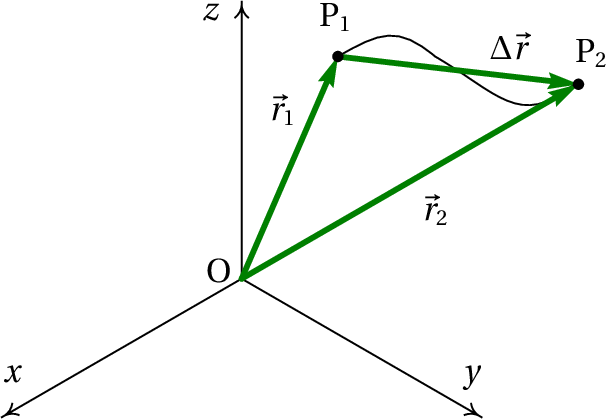Figure 2.6: Trajectory of a point and displacement ∆  r between two instants t 1 and t 2 .

The vector obtained by dividing the displacement by is the average velocity, which has the same direction as the displacement . The velocity vector at a moment is defined as the limit of the average velocity, when the time interval , approaches zero, starting at time

(2.7)

Since the rectangular components of the displacement vector are , and , then the velocity vector is equal to

(2.8)

Applying equation 1.5 to each of the three components of the position vector, the three results can be combined into the following vector equation

(2.9)

The increase of the velocity vector, , in the time interval divided by that time interval defines the acceleration vector

(2.10)

and its rectangular components are the derivatives of the components of the velocity vector

(2.11)

The results obtained from equation 1.11 for each of the three components of the velocity vector can also be combined into a single vector equation

(2.12)

Equations 2.8 and 2.11 are the kinematic equations in 3 dimensions, written in vector form. Since the equality of two vectors implies equality of their components, those two equations are equivalent to , and similar equations for the and components. Therefore, a motion in three dimensions is always the superposition of three one-dimensional motions along the , and axis.

There is also another kinetic equation for each rectangular component, which relates the acceleration with the velocity and the position

(2.13)

those three equations can also be combined into a single vector equation: , where the dot "·" denotes scalar product among vectors, which will be introduced in the next section. However, in order to solve differential equations using the method of separation of variables used in the previous chapter, it will be more useful to solve each of the three equations 2.13 separately.

The speed mentioned in the previous chapter is the magnitude of the velocity vector ; is the component of the velocity along the trajectory, which can be either positive or negative. When vectors are used to describe the motion, and are simply called velocity and acceleration.

### Example 2.1

the velocity of a particle as a function of time is given by the expression (in SI units):

The position of the particle at = 0 is (2  + 5  ). Find the position vector, the velocity and the acceleration at = 15 s and as approaches infinity. Make the plot of the particle's trajectory during the first 60 seconds.

Solution. The components of the velocity can be saved into a list in Maxima

(%i1) v: [5-t^2*exp(-t/5), 3-exp(-t/12)];
(%o1)

And functions diff and integrate can be used to differentiate and integrate that list, in order to find the components of the acceleration and the position. The two components of the acceleration (derivative of the velocity with respect to time) are found in the following way

(%i2) a: diff (v, t);
(%o2)

The components of the position vector are obtained using Equation 2.9.

(%i3) assume (t > 0)\$
(%i4) r: expand([2,5] + integrate(v, t, 0, t));
(%o4)

the command assume was used to tell Maxima that is positive; if that command had not been given, Maxima would have asked about the sign of before computing the integral, since the result depends on that sign.

The position, velocity and acceleration after 15 seconds are then

(%i5) float (subst (t=15, r));
(%o5)
(%i6) float (subst (t=15, v));
(%o6)
(%i7) float (subst (t=15, a));
(%o7)

To find the limit of those vectors when time approaches infinity, function limit is used and infinity is denoted by Maxima's symbol inf

(%i8) limit (r, t, inf);
(%o8)
(%i9) limit (v, t, inf);
(%o9)
(%i10) limit (a, t, inf);
(%o10)

That means that the particle attains a constant velocity , moving away to infinity.

To create the plot of the trajectory, the option parametric of Maxima's function plot2d is used. The and components of the position vector must be given separately, and not in a list, because that is the form expected by plot2d. The first element in list r ( component of the position) is obtained with the syntax r [ 1] and the second element ( component) with r[ 2]

(%i11) plot2d ([parametric,r,r], [t,0,60], [xlabel,"x"], [ylabel,"y"]);

The time interval from 0 to 60 was given using the notation [t , 0 , 60 ]. The result is shown in Figure 2.7.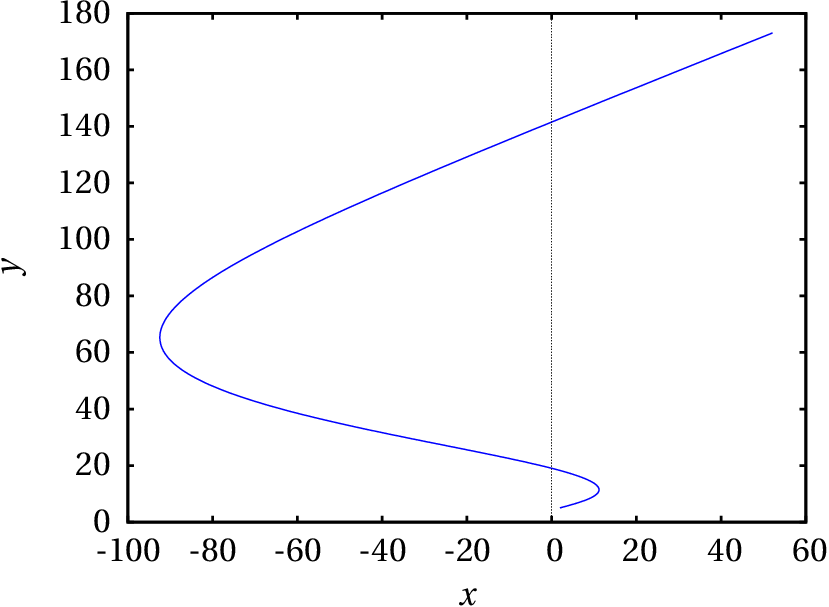Figure 2.7: Trajectory of the particle during the 60 seconds after it passed by the point (5, 2).

### 2.1.3. Scalar product

The dot or scalar product between two vectors and , denoted by placing a dot between the vectors, (to be read as dot ), is defined as the product of their magnitudes and the cosine of the angle between them

(2.14)

Figure 2.8 shows two vectors and and the angle between them. The projection of vector in the direction of vector is equal to and the projection of vector in the direction of vector is equal to . Hence, the dot product of the vectors is equal to the projection of one of them in the direction of the other, times the magnitude of that second vector.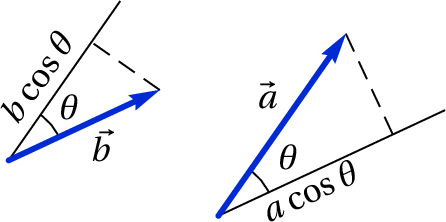Figure 2.8: Two vectors  a and  b and the angle θ between them.

The name scalar is due to the fact that, since the magnitude of the vectors and the angle between them are both scalar quantities, the dot product gives also a scalar quantity, independent of the reference frame chosen.

Two straight lines crossing at a point define two different angles, and (180° − ). In the case of vectors there is no ambiguity in the definition of the angle between them, because if the two vectors are placed on the same initial point, the angle between them is always measured in the region where + is located (see Figure 2.9).

The dot product of two vectors with magnitudes and will always be within the interval [ , ]. If the angle between the vectors is acute, then > 0 and the dot product is positive. If the angle is obtuse, then < 0 and the dot product is negative. If the vectors are perpendicular, then = 0 and their dot product is zero (see Figure 2.9). The minimum value of the dot product, , is obtained when the directions of the vectors are opposite and the maximum value, , is obtained when both vectors are in the same direction.Figure 2.9: Vectors that form acute, right and obtuse angles between them.

Since unit vectors have magnitude equal to 1, the dot product between two unit vectors gives the cosine of the angle between their directions. Thus, the angle between two directions in space can be obtained as the arc cosine of the dot product of two unit vectors in those directions

(2.15)

In terms of the rectangular components of the vectors, the dot product is,

(2.16)

Using the distributive law of the dot product and the fact that the dot product between two different rectangular unit vectors is zero, because they are perpendicular, while the dot product of any unit vector with itself is 1, because its magnitude is 1, a very useful expression is obtained for the dot product

(2.17)

The rectangular components of the two vectors are different in different reference frames, but their dot product ( + + ) must give the same result in any reference frame, since is a scalar quantity.

Using expressions 2.14 and 2.17 to compute the dot product of a vector with itself, the following relation is obtained

(2.18)

It is then concluded that the magnitude of a vector with components ( , , ) is given by

(2.19)

## 2.2. Relative motion

Figure 2.10 shows the position vectors and of two points P and Q at the same instant. The vector is the position of point P relative to Q. The three vectors are related by the following vector equation:

(2.20)

If both points are moving, the three vectors will be continuous functions of time .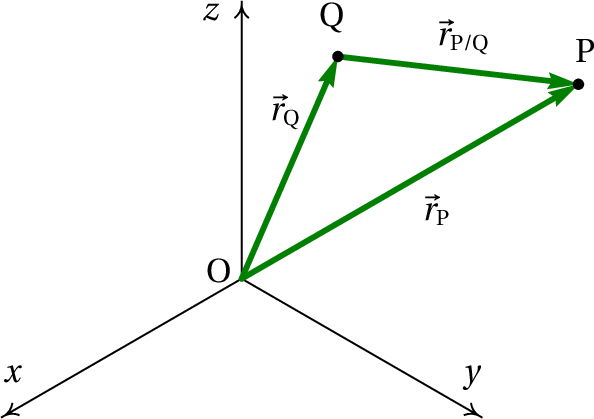Figure 2.10: Position vectors of two points P and Q and position of P relative to Q..

The velocity vectors of the two points are the time derivatives of their position vectors

(2.21)

And the time derivative of the position vector of P relative to Q gives the velocity vector of P relative to Q:

(2.22)

Therefore, differentiation of the three vectors in equation 2.20 with respect to time leads to the relation among the 3 velocities

(2.23)

Namely, the velocity of point P is equal to its velocity relative to another point Q, plus the velocity of that point Q. Also, the velocity of P relative to Q equals the velocity of P minus the velocity of Q.

The relation among the velocities can be differentiated again to obtain a similar expression for the relative acceleration vector

(2.24)

For example, when one is traveling in a train moving with velocity and observes an object with velocity as measured inside the train, the velocity of that object relative to the Earth is equal to + . But since the Earth is moving relative to the Sun with velocity , the velocity of that object relative to the Sun is . Relative to the Galaxy, one would have to add the velocity of the Sun relative to the Galaxy, and so on.

The law of addition of relative velocities and accelerations is used to train the future astronauts. If the trainee is falling freely inside an airplane, his acceleration relative to the Earth is the vector , pointing towards the center of the Earth and with magnitude equal to the acceleration of gravity. If the airplane is also falling freely to the Earth, its acceleration relative to Earth is the same vector (see Figure 2.11). The acceleration of the trainee relative to the airplane is equal to the difference of those two accelerations, which gives zero. That is, relative to the airplane the trainee does not accelerate in any direction but floats inside the plane during the seconds that the airplane pilot can manage to let the plane fall freely.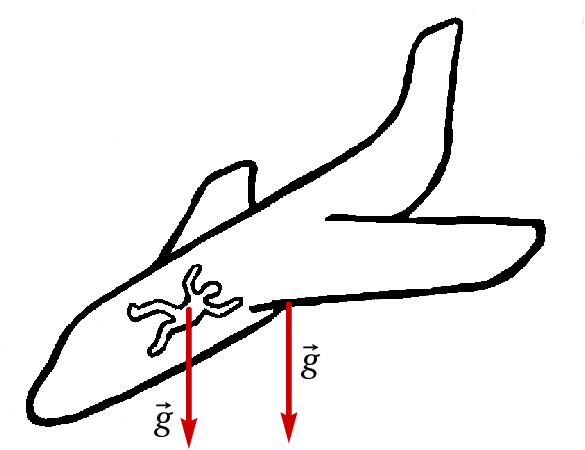Figure 2.11: Airplane and passenger in free fall (zero relative acceleration).

## 2.3. Projectile motion

The motion of an object in free fall, when the air resistance can be neglected, was studied in chapter 1. The same problem will be discussed here, using vectors. If the axis is chosen in the vertical direction and pointing upwards, the vector that represents the acceleration of gravity is

(2.25)

where is approximately 9.8 m/s2.

If a projectile is launched with an initial velocity , the change of the velocity due to the acceleration of gravity is in the opposite direction of , leading to a new velocity that will be in the same plane of the vectors and . It is then concluded that the trajectory of the projectile is always on the vertical plane defined by and . The only exception is when is in the vertical direction, in which case and do not form a plane and the trajectory is a vertical straight line.

### Example 2.2

A cannon shoots a bullet from the roof of a building from the position (in SI units):

and with initial velocity (SI units):

where the axis points upward in the vertical direction and with origin at the ground level. Assuming that the air resistance is negligible, find the maximum height reached by the bullet and the position where it hits the ground.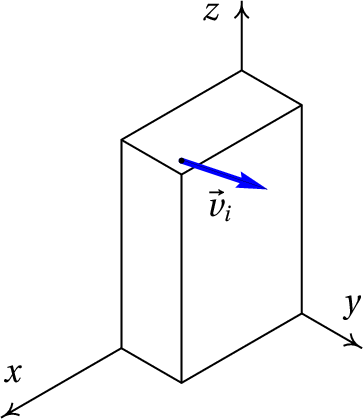Solution. The expression for the velocity as a function of time is found replacing the initial velocity and the expression 2.25 for the acceleration of gravity into equation 2.12 and integrating with respect to time

Where is measured from the moment when the bullet is shot.

The expression for the position vector is obtained substituting this expression into equation 2.9 and evaluating the integral

The maximum height will be reached when the velocity becomes horizontal, namely, when the component of the velocity vanishes

the component of the position vector at that instant gives the maximum height

The instant when the bullet hits the ground is when the component of the position vector vanishes

and the position of the bullet at that moment is

## 2.4. Dependent motions

Some systems which apparently require several variables to describe the motion of its parts, can lead to fewer degrees of freedom due to constraints on those variables. Figure 2.12 shows an example; while the cylinder moves down, the cart moves on the table.

The motion of the cart can be described by the variation of the horizontal distance to the center of the fixed pulley. The motion of the cylinder is the same as the motion of the moving pulley, which means that it can be described by the variation of the vertical distance between the centers of the pulleys.

As long as the string remains stretched without breaking, there will be a relation between the velocities and accelerations of the cylinder and the cart. To find that relation, the expression for the length of the string, , is written in terms of the two distances that are changing, and

(2.26)

where and are the radii of the two pulleys. The string is in contact with one fourth of the perimeter of the fixed pulley ( ) and with half of the perimeter of the moving pulley ( ). Keeping in mind that , , and remain constant and differentiating both sides of this last equation with respect to time, the following result is obtained

(2.27)

That is, the speed of the cart will always be twice as great as the speed of the cylinder. The negative sign means that if the cylinder goes up ( decreases), the cart moves to the left ( increases) and the other way around.

Differentiating once more this last equation, with respect to time, it is found that the tangential acceleration of the cart is also twice as great as the tangential acceleration of the cylinder and with opposite sign

(2.28)

Those relations among the positions, velocities and accelerations of the two objects imply that the system has only one degree of freedom. Once the expressions for the position, velocity and acceleration of one of the objects are know, the corresponding expressions for the other object are obtained by multiplying or dividing by −2.

A second example, with two degrees of freedom, is the system of three pulleys and three cylinders shown in Figure 2.13. The heights of the three cylinders are determined from the three distances , and . Since there is only one string in motion, there will be only one constraint (the length of that string remains constant) which can be used to express one of the 3 distances in terms of the other two and, therefore, the system has two degrees of freedom.

The length of the string is

(2.29)

where the constant is equal to the sum of half of the perimeters of the pulleys, which do not have to be known in order to find the relations among the velocities or accelerations, since it will vanish when the derivatives are computed.

Differentiating both sides of the last equation leads to

(2.30)

In this case there are several possible motions; for example, if cylinder A is moving up and cylinder C is moving down with the same speed, then cylinder B will remain at rest. Or one of the cylinders can be moving down while the other two are moving up. However, it would not be possible that the three cylinders move all up or all down.

Differentiation of equation 2.30 with respect to time leads to the relation among the accelerations

(2.31)

### Example 2.3

In the system shown in the figure, find the speed of the upward motion of the cylinder when the ring A is pulled down with speed equal to 2 m/s.Solution. In this case there are 4 parts with different motions, the three moving pulleys and ring A (the motion of the cylinder is the same as that of the moving pulley where it is hung) and 3 strings with constant lengths. Hence, this system has only one degree of freedom and the speed of A given will make it possible to find the velocities of all the other parts.

If is the distance from the ceiling to the ring and , and the distances from the ceiling to each of the moving pulleys, as shown in the figure, the lengths of the three strings are

Differentiating both sides of each of these equations, the results are

and solving for in terms of ,

that is, the speed of the downward motion of the ring is 8 times as great as the speed of the upward motion of the cylinder. Therefore, the cylinder moves up with a speed of 0.25 m/s.

## Questions

1. The block in the figure is on an incline with a 40° angle with the horizontal. One end of the string is attached to the wall, while the other end is being pushed with speed in the direction shown in the figure. What is the correct expression for the speed of the block in terms of ?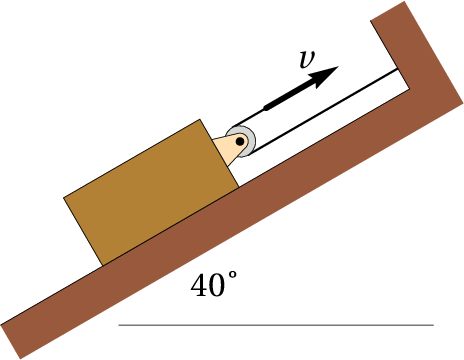2. A car enters a curve with speed equal to 10 m/s in the South direction and 6 seconds later it still has the same speed but in the West direction. Find the magnitude of the average acceleration in that interval of time.
1. 1.67 m/s2
2. 2.36 m/s2
3. 2.89 m/s2
4. 3.33 m/s2
5. 0
3. A projectile is thrown with an angle of 40° over the horizontal. If the speed of the projectile when it reaches its maximum height is 80 m/s and the air resistance can be neglected, what was the approximate value of the speed at which it was thrown?
1. 104.4 m/s
2. 124.5 m/s
3. 61.3 m/s
4. 51.3 m/s
5. 80 m/s
4. A particle moving at 4 m/s in the direction of the axis is given a constant acceleration of 3 m/s2, in the direction of the axis, during 2 seconds. Find the final speed of the particle after those 2 seconds.
1. 5.0 m/s
2. 6.3 m/s
3. 7.2 m/s
4. 8.4 m/s
5. 10.0 m/s
5. In the system shown in the figure, with a cart, a bar, a cylinder, 2 moving pulleys and 4 fixed pulleys, the bar remains always in a horizontal position. How many degrees of freedom does this system have?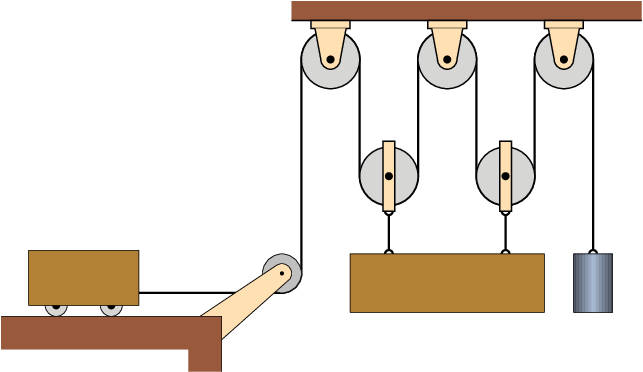1. 1
2. 2
3. 3
4. 4
5. 5

## Problems

1. (a) Prove the law of cosines for plane triangles: any plane triangle with sides of lengths , and , satisfies the following equation
where is the angle opposite to the side of length ; The Pythagorean theorem is a particular case when is a right angle. Hint: draw the triangle formed by the two vectors and and their sum and compute the dot product . (b) The angle between two vectors with magnitudes equal to 5 and 8 units is 42°; find the magnitude of the sum of those two vectors using the law of cosines.
2. Given two vectors and , find:
1. The magnitude of each vector.
2. Their dot product .
3. The angle between them.
4. Their sum .
5. Their difference .
3. The velocity of a particle moving on the plane is given by the expression: (SI units). At the initial moment the particle is on the axis with position .
1. Find the moment when it passes through the axis and its distance from the origin at that moment.
2. Compute the acceleration at and at the moment when it passes through the axis.
4. An object is initially at the position (SI units) with velocity and its acceleration at any moment is given by the expression . Find the expressions for the velocity and the position as a function of time.
5. A projectile is thrown from the ground in a direction 30° above the horizontal. Find the initial speed that should be given to the projectile to make it hit the ground 30 m away from the point where it was thrown, if the air resistance can be neglected.
6. A pebble rolls down the roof of a house, which is inclined 20° from the horizontal. At the moment when the pebble leaves the roof and starts falling freely, it has a speed of 4 m/s and it is 6 m above the ground. Assuming that the air resistance is negligible,
1. Find the time it takes to hit the ground, from the moment when it leaves the roof.
2. What is the horizontal displacement of the pebble during its free fall?
3. Compute the angle between the vertical and the velocity of the pebble at the moment when it hits the ground.
7. A boat transports passengers across a river, following the shortest path of 1.5 km between the two river banks. When the boat's motor works at its full power, the trip takes 20 minutes on a day when the speed of the river current is 1.2 m/s. Find the speed of the boat that day: (a) relative to the ground and (b) relative to the water. (c) Find the minimum time that it would take the same trip on a day when the speed of the river current was 0.8 m/s.
8. A passenger standing on a chair of a train that travels with constant velocity of magnitude 35 km/h throws an object horizontally in the direction opposite to the velocity of the train. Relative to the floor of the train carriage, the object is thrown at a height of 3 m and it moves horizontally 3 m before it hits the floor. Find the horizontal distance traveled by the object, relative to the Earth, from the moment it was thrown until it hit the carriage floor.
9. An object moves from the origin at and at its position vector is given by (SI units).
1. What will be the distance from the object to the origin in the limit ?
2. Find the total distance traveled from to (the integral obtained cannot be solved analytically, but it can be computed numerically using Maxima's function romberg, which needs the same 4 arguments as integrate; instead of for the upper limit of the integral, use , obtain the result and gradually increase that value until the result seems to approach a limit).
10.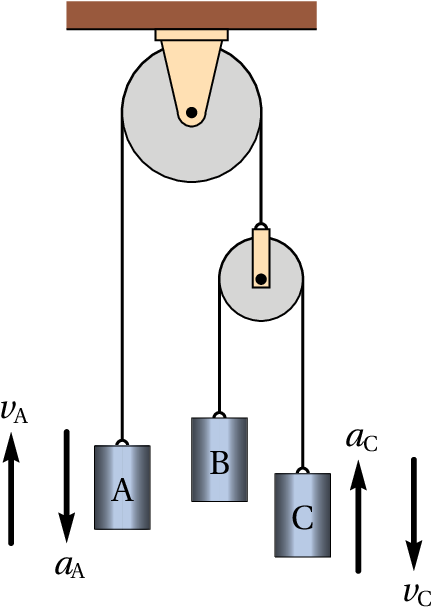Three cylinders A, B and C are hanged by the two-pulley system shown in the figure. At a given instant, the velocity of block A is  m/s, upwards, and its acceleration is  m/s2, downwards; at the same instant, the velocity and acceleration of block C are:  m/s, downwards,  m/s2, upwards. Find the velocity and acceleration of block B, at the same instant, and say whether they are in the upwards or downwards direction.
11. For the system show in the figure, find the relation between the velocities and accelerations of the bar A and the cylinder B, assuming that the bar A only moves up or down, remaining always in a horizontal position.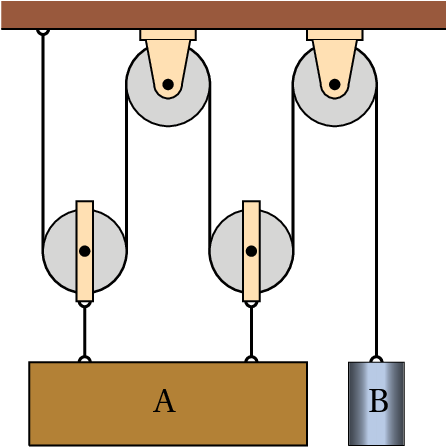12. The cart in the figure moves to the left with constant speed of 4 m/s. The height is 25 cm and is chosen as the moment when the distance é is zero. Find the expressions for the velocity and acceleration of the cylinder (assume that the radii of the pulleys are very small).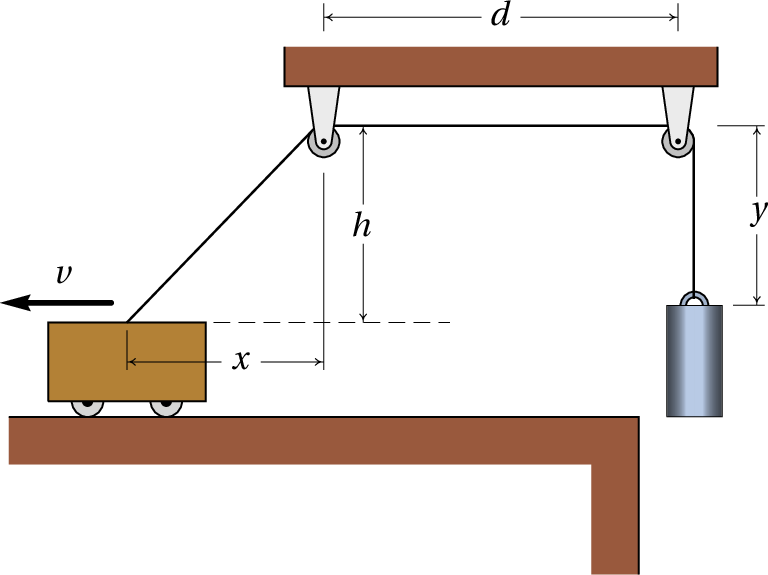Questions: 1. B. 2. B. 3. A. 4. C. 5. B.

Problems

1. (a) . Since the angle between the vectors is , it follows that
(b) 12.18 units.
2. (a) , . (b) −25. (c) 123.5°. (d) . (e) .
3. (a)  s,  m.
(b) At ,  m/s2. When it passes through the axis,  m/s2.

4. = 18.43 m/s.
5. (a) 0.976 s. (b) 3.67 m. (c) 19.0°.
6. (a) 1.25 m/s. (b) 1.73 m/s. (c) 16 minutes and 20 seconds.
7. 4.6 m.
8. (a) 5 m. (b) 5.23 m.
9. 5 m/s downwards and acceleration equal to zero.
10. ,
11.           (SI)
Wrong

The block and the end of the string cannot have the same speed because the distance between them is increasing.

(click to continue)

Correct

For each centimeter of string being pulled up, the center of the pulley will move up 1/2 cm on the incline.

(click to continue)

Wrong

The angle does not matter, because an increase or decrease of that angle does not change the relation between the motions of the block and the string.

(click to continue)

Wrong

The speed of the block must be smaller than the speed of the end of the string because the distance between them is increasing.

(click to continue)

Wrong

The angle does not matter, because an increase or decrease of that angle does not change the relation between the motions of the block and the string.

(click to continue)

Wrong

The change of the velocity is not 10 m/s, but rather the hypotenuse of a right triangle where the other two sides are the initial and final speeds.

(click to continue)

Correct

(click to continue)

Wrong

The change of the velocity is the hypotenuse of a right triangle where the other two sides are the initial and final speeds.

(click to continue)

Wrong

The change of the velocity is the hypotenuse of a right triangle where the other two sides are the initial and final speeds.

(click to continue)

Wrong

The change of the velocity is not 0, because the velocity is a vector and the initial and final velocity vectors are not equal because their directions are different.

(click to continue)

Correct

(click to continue)

Wrong

The speed is the hypotenuse of a right triangle with one the other sides equal to the vertical component of the velocity and the other side equal to the horizontal component of the velocity, which remains constant. At the point of maximum height, the vertical side of that triangle is zero and the horizontal projection of the velocity is equal to the speed. At the moment when the projectile is thrown, the angle between the hypotenuse and the horizontal side is 40°.

(click to continue)

Wrong

The speed is the hypotenuse of a right triangle with one the other sides equal to the vertical component of the velocity and the other side equal to the horizontal component of the velocity, which remains constant. At the point of maximum height, the vertical side of that triangle is zero and the horizontal projection of the velocity is equal to the speed. At the moment when the projectile is thrown, the angle between the hypotenuse and the horizontal side is 40°.

(click to continue)

Wrong

The speed is the hypotenuse of a right triangle with one the other sides equal to the vertical component of the velocity and the other side equal to the horizontal component of the velocity, which remains constant. At the point of maximum height, the vertical side of that triangle is zero and the horizontal projection of the velocity is equal to the speed. At the moment when the projectile is thrown, the angle between the hypotenuse and the horizontal side is 40°.

(click to continue)

Wrong

The speed is the hypotenuse of a right triangle with one the other sides equal to the vertical component of the velocity and the other side equal to the horizontal component of the velocity, which remains constant. At the point of maximum height, the vertical side of that triangle is zero and the horizontal projection of the velocity is equal to the speed. At the moment when the projectile is thrown, the angle between the hypotenuse and the horizontal side is 40°.

(click to continue)

Wrong

During the 2 seconds the acceleration leads to a velocity component equal to 6 m/s.

(click to continue)

Wrong

The final speed is the hypotenuse of a right triangle with the other sides equal to and . There will be a component due to the acceleration .

(click to continue)

Correct

(click to continue)

Wrong

The final speed is the hypotenuse of a right triangle with the other sides equal to and . There will be a component due to the acceleration .

(click to continue)

Wrong

The acceleration leads to a velocity increase of 6 m/s, but since that increase is in the x direction it cannot be added directly to the initial velocity, which is in the direction. The sum must be done as a sum of vectors.

(click to continue)

Wrong

There are three objects with different motions: the cart, the bar and the cylinder (the motion of the moving pulleys the same as that of the bar) and only one constraint: the length of the string remains constant.

(click to continue)

Correct

There are three objects with different motions: the cart, the bar and the cylinder (the motion of the moving pulleys the same as that of the bar) and only one constraint: the length of the string remains constant.

(click to continue)

Wrong

There are three objects with different motions: the cart, the bar and the cylinder (the motion of the moving pulleys the same as that of the bar) and only one constraint: the length of the string remains constant.

(click to continue)

Wrong

There are three objects with different motions: the cart, the bar and the cylinder (the motion of the moving pulleys the same as that of the bar) and only one constraint: the length of the string remains constant.

Wrong

There are three objects with different motions: the cart, the bar and the cylinder (the motion of the moving pulleys the same as that of the bar) and only one constraint: the length of the string remains constant.

(click to continue)